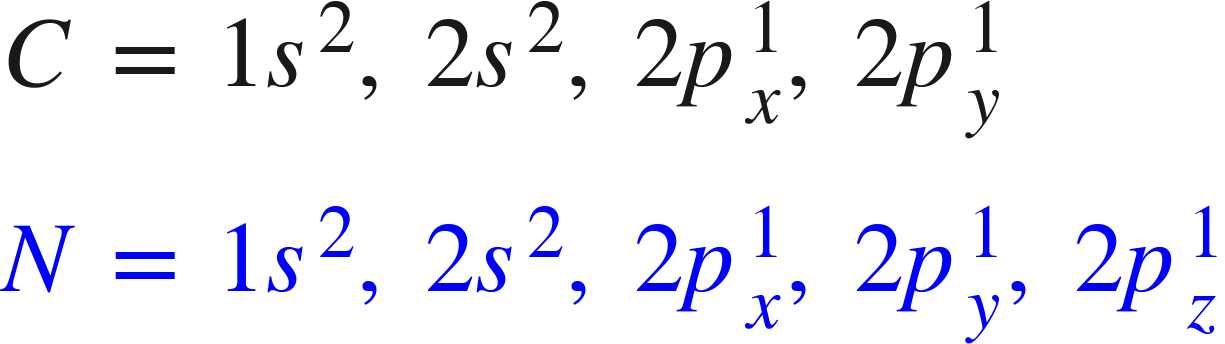# Problem: Using the molecular orbital model, write electron configurations for the following diatomic species and calculate the bond orders. Which ones are paramagnetic? Place the species in order of increasing bond length and bond energy.a. CN + b. CN c. CN -

###### FREE Expert Solution

To calculate bond order of CN+, CN and CN- and predict other properties, we will start by drawing the molecular orbital diagram for all three.

CN+ is composed of two atoms from 2nd period. The electronic configurations of C and N are:Carbon is a group 4A element having 4 valence electrons and nitrogen has 5 valence electrons (Group 5A). There is 1 positive charge, so we will subtract 1 electron from the total for the positive charge.

Total valence electrons are 4+5-1 = 8 e-.

80% (356 ratings)###### Problem Details

Using the molecular orbital model, write electron configurations for the following diatomic species and calculate the bond orders. Which ones are paramagnetic? Place the species in order of increasing bond length and bond energy.

a. CN +

b. CN

c. CN -